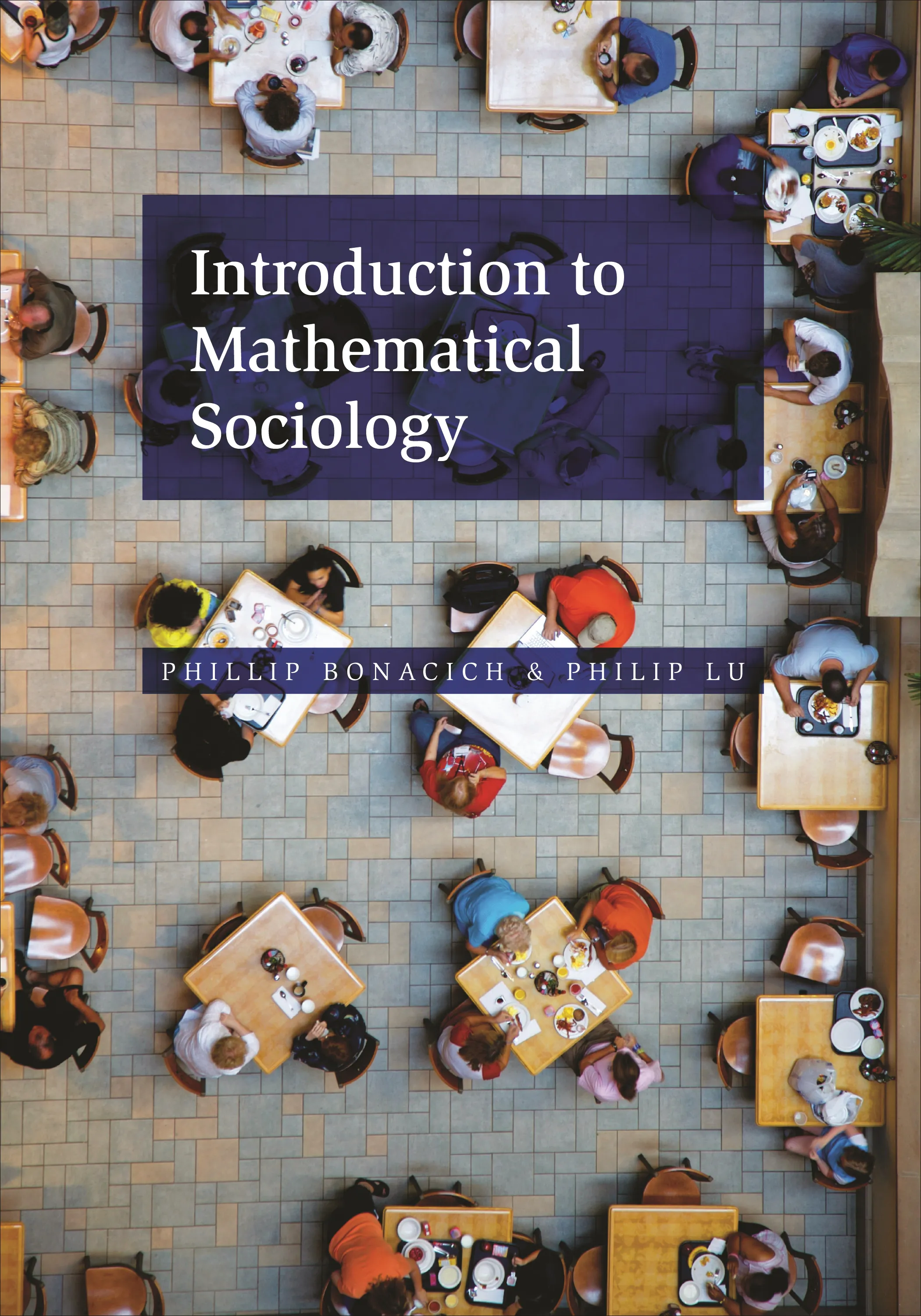Phillip Bonacich

# Introduction to Mathematical Sociology

A comprehensive textbook on the tools of mathematical sociology and their applications## Hardcover

Price:
\$58.00/£48.00
ISBN:
Published:
Apr 1, 2012
2012
Pages:
240
Size:
7 x 10 in.
Illus:
25 halftones. 58 line illus. 30 tables.

Mathematical models and computer simulations of complex social systems have become everyday tools in sociology. Yet until now, students had no up-to-date textbook from which to learn these techniques. Introduction to Mathematical Sociology fills this gap, providing undergraduates with a comprehensive, self-contained primer on the mathematical tools and applications that sociologists use to understand social behavior.

Phillip Bonacich and Philip Lu cover all the essential mathematics, including linear algebra, graph theory, set theory, game theory, and probability. They show how to apply these mathematical tools to demography; patterns of power, influence, and friendship in social networks; Markov chains; the evolution and stability of cooperation in human groups; chaotic and complex systems; and more.

Introduction to Mathematical Sociology also features numerous exercises throughout, and is accompanied by easy-to-use Mathematica-based computer simulations that students can use to examine the effects of changing parameters on model behavior.

• Provides an up-to-date and self-contained introduction to mathematical sociology

• Explains essential mathematical tools and their applications

• Includes numerous exercises throughout

• Features easy-to-use computer simulations to help students master concepts